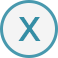# 九月鹿港家族遊(((林保君你居然在偷喝飲料)))easy小媽順手給保君啃啃雞腿，easy小媽不管保君是不是看的懂書，(((浩浩蕩蕩的一行人~我們的背影)))easy小媽最愛她們這種探索大自然的感覺，(((一樣都是排行老二的保君和于嫻阿姨)))easy小媽和權倫舅舅小時候長超像的，(((外公想在這地方和大家拍拍照)))(((鄭式夫妻一家三口和後面黑色的車車)))(((很愛和保君拍個照)))(((這邊只開到4點半就關門)))(((保君真的很愛黏人，樣子真撒嬌)))(((等飲料的空檔還是要拍個照)))(((什麼都可以玩~)))(((什麼都可以拍~)))((林保君還是在偷喝飲料)))(((鹿港~好地方)))(((林香均你很有演戲的天份喔)))(((逛街去囉~)))(((日記篇同步播映)))

### 編輯推薦### 儲存至我的收藏### 新增收藏分類